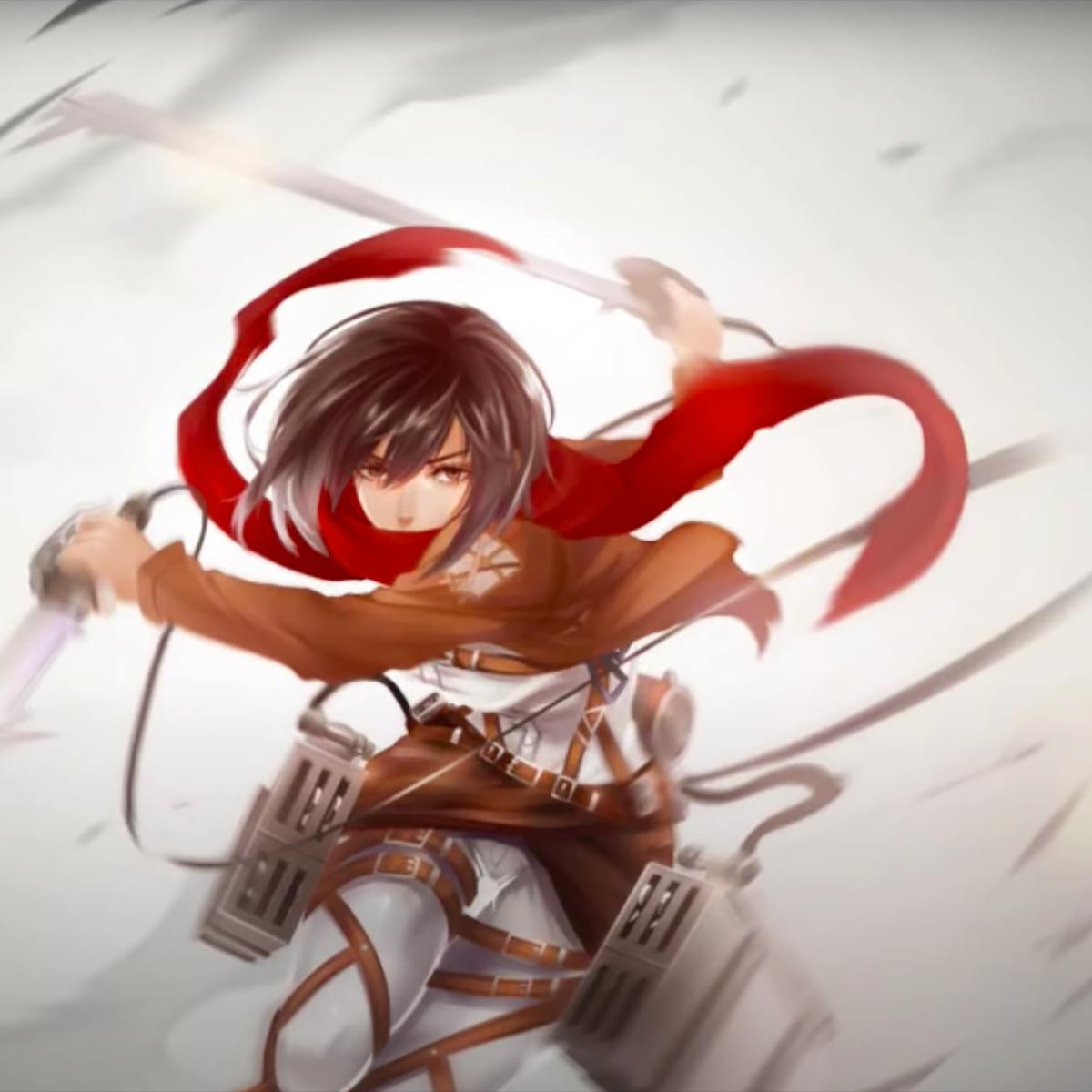# Conversation Heart (Noragami)

4 December 2020
7
02:07
-5
• ##### TEMPO
71
0
(0)

f g [uspih]|[ypi]| |[yuoha]|[usoe]|f g [sife] d [pi] [yw] [si]|[fa8] [wp] [yua]|f g [upih]|[spi]|l|[skf6]0| |[wj] h [usjfQ] k [rphD]|G|[pfa0] r u|f g [uspih]|[ypi]| |[yuoha]|[usoe]||h [sig] e|d [ywpi] s [ufa8] w [yp]|f g [upih]|[spi]|l|[uokfe]| |[wj] h [usjfQ] k [rhD]|G f [urpf0]|O| |[of8] w [uof] w [uof] w [pif8] e i d [yf] [ge] [ohd0] y o y [oha] y [wsfa] T u P o|[ypd] p|fdS[qd] [tf] [og0] t [of] t [I(] t [si9] e [ui] e [ui]|[si5] 9 e y i p [of8] w [of] w [uof] w [of0] r I r o r [wf] y o ygfd[fP] [yg] [heS] u g u [pfS]|[pdQ] y p yfdS[qd] [tf] [og0] t [of] t [I(] [td] [si9] e [usi] e [wsi] y [us] w|u o s [ujQ]|j kjHj k [rplD]|[ok]||j [uhdE]|h|[wuhP]|[ogT]| |e|[dQ] y [pd] yfdS[qd] [tf] [og0] t [of] t [I(] [td] [si9] e [usi] e [wsi]|[us] w u|f g [uspih]|[ypi]| |[yuoha]|[usoe]|f g [sif] [ed] [pi]|[ywsi]|[fa8] [wp] [yua]|f g [uspih]|[spi]|l|[skf6]0| |h f [ied] f [wpi]|5|[us8] w [uo]

Rate This Music Sheet:

Average rating 0 / 5. Vote count: 0

No votes so far! Be the first to rate this music sheet.

Thank you for rating this song!

If you have any specific feedback about how to improve this music sheet, please submit this in the box below.

## About This Music Sheet

Conversation Heart (Noragami) is a song by Taku Iwasaki. Use your computer keyboard to play Conversation Heart (Noragami) on Virtual Piano. This is an Intermediate song and requires a lot of practice to play well. The recommended time to play this music sheet is 02:07, as verified by Virtual Piano legend, Arda. The song Conversation Heart (Noragami) is classified in the genres: Manga, Songs From TV, Japan on Virtual Piano. You can also find other similar songs using Anime.

## Related

•DDGg SSD DGg S|| J GH g G D g S J GH g G D g S L jk H j G H f L jk H j G H f IafjgsO g SD s S P s O g SD s S s P O g SD s S P s O g SD s S s P O g SD s S P s O g SD s S s P O g SD s S P s O g SD s S s P O P PPP sSsPp|ip P iPs isS iSD D DDDP D SSSO S D DDD P D D dd| D DGg SSD DGg S D DGg D D S P S D Gg SSD DGg S DDGg DS P J|H| D DGg SSD DGg S D DGg D D S P S D DGg S DDGg SD Gg DS D|| J GH g G D g S L jk H j G H f L jk H j G H f L jk H j G H f L jk H j G H f x lz k l j k h x lz kl||xvb
Level: 2
Length: 01:06
Easy

#### Linked Horizon

•[uth] f d s d f [uth] f d s d f [uth] f h [tje] f j [uth] f d s|| [o80] u y t y u [o80] u y t y u [o80] u o [pe] u p [aW0] O I u|| [aW0] O I u I O [aW0] O I u I O [aW0] O a [s8] O s [aW0] O I u|| [aW0] O I u I O [aW0] O I u I O [aW0] O a [se] p s [wrd] a p o|| [wrd] a p o p a [wrd] a p o p a [wrd] a o y r w d a o [wri]|| [uth] f d s d f [uth] f d s d f [uth] f h [tje] f j [yji] g f d|| [qp9] i u y u i [qp9] i u y u i [p9] i p [wa] i a [a0] o a [s8] o s [sq] p s [d9] p d [f0] W r 0 W r [qd] e t [sq] e t [a0] W r 0 W r [wa] r y w|| [o80] u y t y u [o80] u y t y u [o80] u o [pe] u p [o80] y t|| [h8] f d [se] d f [h8] f d [se] d f [h8] f d [se] d f [sh80]
Level: 6
Length: 01:38
Intermediate

#### Edvard Grieg

•l k l z|l z c|x z l|| j|k|l|z|l k l z|l|l|k l z|l|x| c x|z|l|| l|k|j|g|h|j|l|k j|k|ll k l h g f s| f f g h h| g f|s|d|| d d f g g||d|a|s|| s|j|h|g|f|s|d| f f|| g|f|g| g|| g g g f| d|s d|h|| g h l|f|d|s| g||f||d|| s|s|f|| gfdh|h|h h h h s|s|| h h h h h h h h|s s|d f| d d s d|s f| g|h|j||| k|j|H|| j| kl k l z|h h z|l k l|| l k l z|l z x||| l k l z|h h z|l k l|| x x x z|l k jl k l z|h|z|l k l|| h l k l z|v c x x||| x x x z|l|z|x c x|| h x x x z|l k jl|k|l|k|l|k|j|h|h j h j| h j h j| j|k| l|k|l|k|l|k|l|z|x x x z|l k j
Level: 2
Length: 02:02
Easy

#### Goose House